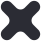Close

Mathematics

Key Stage 3 Mathematics Year 7

 Subject Content Students in year 7 follow the White Rose mastery scheme of learning. The scheme follows on from the KS2 curriculum ensuring students broaden and develop upon these key skills.    Autumn term – Understanding and applying algebraic notation, equality and equivalence, place value and ordering and fractions, decimals and percentages.  Spring term – Solving problems with addition and subtraction, solving problems with multiplication and division, four operations with directed number, addition and subtraction of fractions. Summer term – construction, measuring and geometric notation, developing geometric reasoning, developing number sense, sets and probability, prime numbers and proof. KS3 Fundamental Skills The key fundamentals for mathematics are number, algebra, geometry, statistics and ratio and proportion. Students will apply these throughout the year interleaving topics within each other so that they see and develop an understanding of how each skills links with other areas of maths and other subjects within the curriculum. Background/Extended Learning Use MyMaths to further your understanding.   Discuss at home different application to maths. Each question is related to a real life situations from buying tickets to electricity bills. Reflect on your learning and keep practice the skills. Create knowledge organisers and flash cards. This will help to learn the key facts. The key to success is using the skills regularly.

Year 8

 Subject Content Students in year 8 build on their learning in year 7 ensuring students broaden and develop upon these key skills.    Autumn term – Fractions, decimals and percentages, algebra proficiency, sequences, visualising and construction, proportional reasoning.   Spring term – Calculating risk, numbers and the number systems, calculations, solving equations and inequalities, calculating space and investigating angles.   Summer term – Algebraic techniques, presenting data, calculating data, calculations KS3 Fundamental Skills The key fundamentals for mathematics are number, algebra, geometry, statistics and ratio and proportion. Students will apply these throughout the year interleaving topics within each other so that they see and develop an understanding of how each skills links with other areas of maths and other subjects within the curriculum. Background/Extended Learning Use MyMaths to further your understanding.   Discuss at home different application to maths. Each question is related to a real life situations from buying tickets to electricity bills.  Reflect on your learning and keep practice the skills. Create knowledge organisers and flash cards. This will help to learn the key facts. The key to success is using the skills regularly.

Year 9

 Subject Content Students in year 8 build on their learning in year 7 ensuring students broaden and develop upon these key skills.    Autumn term – Fractions, decimals and percentages, algebra proficiency, sequences, visualising and construction, proportional reasoning. Spring term – Calculating risk, numbers and the number systems, calculations, solving equations and inequalities, calculating space and investigating angles. Summer term – Algebraic techniques, presenting data, calculating data, calculations. KS3 Fundamental Skills The key fundamentals for mathematics are number, algebra, geometry, statistics and ratio and proportion. Students will apply these throughout the year interleaving topics within each other so that they see and develop an understanding of how each skills links with other areas of maths and other subjects within the curriculum. Background/Extended Learning Use MyMaths to further your understanding.   Discuss at home different application to maths. Each question is related to a real life situations from buying tickets to electricity bills.  Reflect on your learning and keep practice the skills. Create knowledge organisers and flash cards. This will help to learn the key facts. The key to success is using the skills regularly.

Key Stage 4

 Course Name GCSE mathematics Exam Board Edexcel Assessment Detail 100% exam based assessment.  3 papers. Each paper last 1 ½ hours.   Students will either complete the higher tier (grades 4 to 9) or foundation tier (grade 1 to 5) Web Link to specification Specification Subject Content Students will develop knowledge and fluency to apply skills involving number, algebra, ratio and proportion and rates of change, geometry and measure and statistics and probability.  For students following the foundation tier, approximately 50% of the contents applies to number and ratio, proportion and rates of changes.  For students following the higher tier, approximately 30%of the content applies to algebra. Skills Taught Number   Calculations, fractions, decimals and percentages, measure and accuracy,   Algebra   Notation, vocabulary and manipulation, graphs, solving equations and inequalities, sequences  Ratio, proportion and rate of change  Proportionality, ratio and links to percentages and fractions  Geometry and measure  Properties and construction, mensuration and calculations, vectors  Probability and statistics  Measures to describes data, diagrams to display data and calculating probability in a variety of contexts Background/Extended Learning Use Mymaths and GCSE pod to further your understanding.   Mathsgenie.co.uk provide revision videos, past exam questions and solutions. Discuss at home different application to maths. Each question is related to a real life situations from buying tickets to electricity bills.